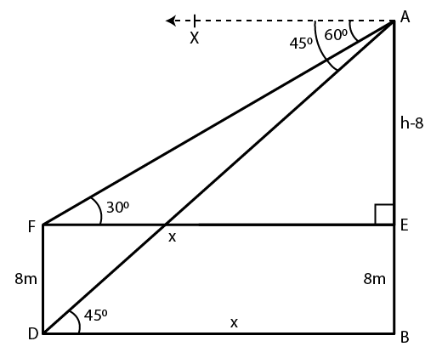Guru

# The angles of depression of the top and the bottom of an 8 m tall building from the top of a multi-storeyed building are 30 and 45 respectively. Find the height of tire multi-storeyed building and the distance between the two buildings, correct to two decimal places.

• 0

sir this is the important  question from the book -ML aggarwal( avichal publication) class 10th , chapter20 , heights and distances
The angles of depression of the top and the bottom of an 8 m tall building
from the top of a multi-storeyed building are 30 and 45 respectively.
Find the height of tire multi-storeyed building and the distance between the two buildings,
correct to two decimal places.

question no 32 , heights and distances , ICSE board, ML Aggarwal

Share

1. Consider AB as the height and CD as the building

The angles of depression from A to C and D are 300 and 450

∠ACE = 300 and ∠ADB = 450

CD = 8 m

Take AB = h and BD = x

From the point C

Construct CE parallel to DB

CE = DB = x

EB = CD = 8 m

AR = AB – EB = h – 8tan θ = AB/DB

Substituting the values

tan 450 = h/x

So we get

1 = h/x

x = h

In right triangle ACE

tan 300 = AE/CE

Substituting the values

1/√3 = (h – 8)/ h

By further calculation

h = √3h – 8√3

So we get

√3h – h = 8√3

h (√3 – 1) = 8√3

h = 8√3/(√3 – 1)

Multiply and divide by √3 + 1

h = 8√3/ (√3 – 1) × (√3 + 1)/ (√3 + 1)

h = 8 (3 + √3)/ (3 – 1)

Here

h = 8 (3 + 1.732)/ 2

h = 4 × 4.732

h = 18.928

h = 18.93 m

x = h = 18.93 m

Here

Height of multi-storeyed building = 18.93 m

Distance between the two buildings = 18.93 m

• 0